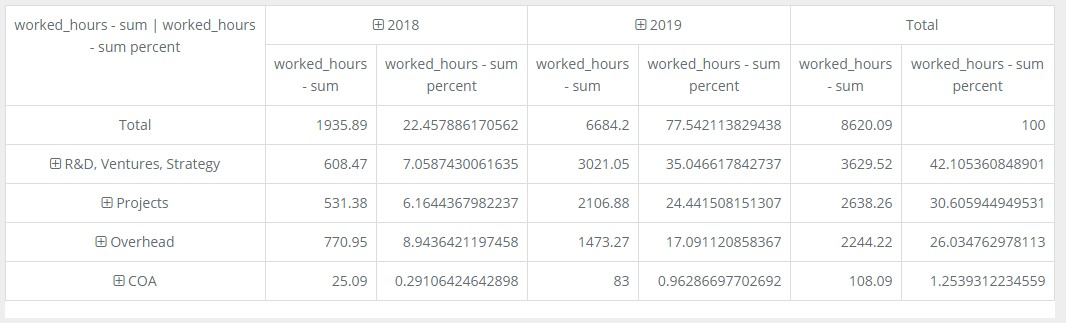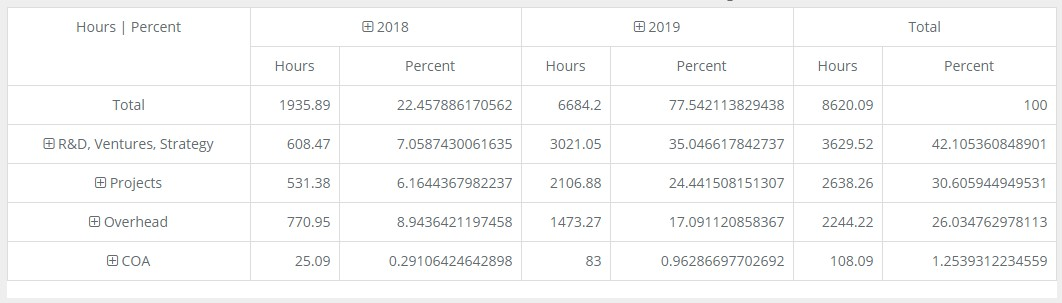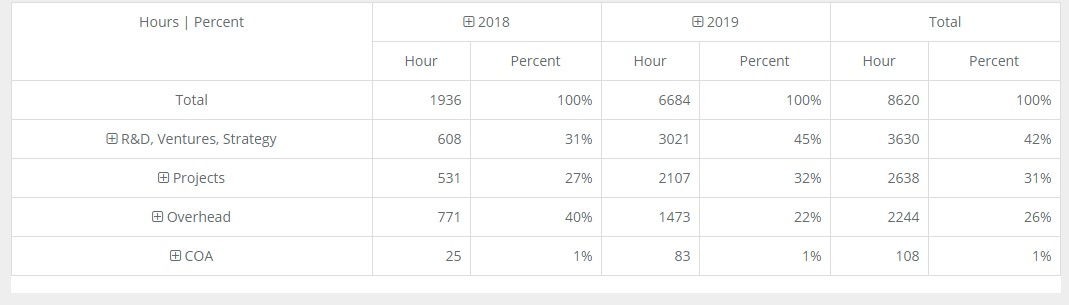# KoolReport's Forum

Official Support Area, Q&As, Discussions, Suggestions and Bug reports.

# PivotTable value formatting. #905

Daan Schoofs opened this topic on on Jun 5, 2019 - 8 comments

Daan Schoofs commented on Jun 5, 2019

Hello,

I'm trying to format my PivotTable values. I've tried to use a ColumnMeta before piping the data throught the Pivot process and I've tried to use dataMap to format the values. Unfortunately I remain unsuccesful.I'm want to round all values to whole numbers (without decimals), add a % suffix to "%_hours - sum percent" and change the datafield names to "hours" and "percent".

This is my piping process:

``````        \$this->src("flatModel")
->pipe(new OnlyColumn(array(
"project.duodeka_type_name",
"project.customer.name",
"date",
"worked_hours",
)))
->pipe(new copyColumn(array(
"date2" => "date",
"%_hours" => "worked_hours"
)))
->pipe(new TimeBucket(array(
"date" => "year",
"date2" => "quarter"
)))
->pipe(new Group(array(
"by" => array("project.duodeka_type_name", "project.customer.name", "date", "date2"),
"sum" => "worked_hours"
)))
->pipe(new ColumnMeta(array(
"worked_hours" => array(
"type" => "number",
"decimals" => 0,
"thousandSeparator" => ".",
"decimalPoint" => ",",
),
"%_hours" => array(
"type" => "number",
"decimals" => 0,
"suffix" => "%"
),
"project.duodeka_type_name" => array(
"align" => "left"
),
"project.customer.name" => array(
"align" => "left"
)
)))
->pipe(new Pivot(array(
'dimensions' => array(
'row' => 'project.duodeka_type_name, project.customer.name',
'column' => 'date, date2'
),
'aggregates' => array(
'sum' => 'worked_hours',
'sum percent' => '%_hours'
)
)))
->pipe(\$this->dataStore("duodeka_cockpit")); // cockpit. main pivot table
``````

And here's my view:

`````` PivotTable::create(array(
"dataStore"=>\$report->dataStore("duodeka_cockpit"),

"rowDimension"=>"row",
"rowSort" => array("worked_hours - sum" => "desc",),
"rowCollapseLevels" => array(0),

"columnDimension"=>"column",
"columnSort" => array("year, quarter" => "asc"),
"columnCollapseLevels" => array(0),

"measures"=>array(
"worked_hours - sum",
"%_hours - sum percent"),

"width" => "100%",
"template"=>"PivotTable-Bun",
));

``````

Oh and another question: I would like to see a total per year, instead of a full total of the table. right now the total is split between 2018 and 2019. How can I achieve this?

Kind regards,

Wessel

David Winterburn commented on Jun 5, 2019

Hi Wessel,

1 . You wouldn't need to set column meta for the sum percent field because the Pivot process would do that for you ('decimals' => 2, 'suffix' => '%').

2 . I think you also don't need the Group process before the Pivot process so the code could look like:

``````...
->pipe(new TimeBucket(array(
"date" => "year",
"date2" => "quarter"
)))
->pipe(new Pivot(array(
'dimensions' => array(
'row' => 'project.duodeka_type_name, project.customer.name',
'column' => 'date, date2'
),
'aggregates' => array(
'sum' => 'worked_hours',
'sum percent' => 'worked_hours', //you could use sum percent on the same column
)
)))
->pipe(\$this->dataStore("duodeka_cockpit")); // cockpit. main pivot table
``````

For changing the data field names please try:

``````        PivotTable::create(array(
...
'map' => array(
'dataField' => function(\$dataField, \$fieldInfo) {
\$v = \$dataField;
if (\$v === 'worked_hours - sum')
\$v = 'Hours';
else if (\$v === 'worked_hours - sum percent')
\$v = 'Percent';
return \$v;
},
),
...
));
``````

3 . You can't set css property in column meta. To make the pivot row and column headers align left (which should be default but it seems your css theme sets text-align: center for table td), please add the following css rules to your page:

``````<style>
text-align: left !important;
}
</style>
``````

4 . I'm not sure about your final question but if you expend the column headers 2018 and 2019 you could see their total columns. If you don't want them to collapse by default, please remove this option from your view file:

``````"columnCollapseLevels" => array(0),
``````

Thanks!

Daan Schoofs commented on Jun 5, 2019

Hi David,

thank you for your quick response! changing the names worked perfectly, thank you for your input!

I've also removed the Group and columnMeta process for the datasource (The values haven't changed, which is good).

Unfortunately the value formatting is still off:You can see here that the field names have changed, but there's still decimals in the values and there's no suffix for the percent column. How can I add this? I've tried to use `'dataCell'` in the `'map'` function, but i get a syntax exception for unexpected `'=>'` that I can't place, because I've copied the documentation from Pivot.

``````...
},
'dataCell' => function(\$value, \$cellInfo) {
if(\$cellInfo["fieldName"] === "Percent"){
\$cellInfo["formattedValue"] => round(\$value,0) . "%";
}else if (\$cellInfo["fieldName"] === "hours"){
\$cellInfo["formattedValue"] => round(\$value,0);
}
return \$cellInfo["formattedValue"];
}
)
...

``````

About my final question. You see now that in the total row, the percentage for 2018 is 22% and the percentage for 2019 78%. I want to see the distribution where for each column, the total is 100%. For example:

``````            2018    2019    Total
totals    100%    100%    100%
row1        47%    55%    20%
row2        63%    45%    80%

``````

Kind regards.

David Winterburn commented on Jun 5, 2019

Hi Wessel,

I think you have syntax problem with these commands:

``````\$cellInfo["formattedValue"] => round(\$value,0) . "%";
...
\$cellInfo["formattedValue"] => round(\$value,0);
``````

They should be:

``````\$cellInfo["formattedValue"] = round(\$value,0) . "%";
...
\$cellInfo["formattedValue"] = round(\$value,0);
``````

As for your sum percent per year column problem, it's a bit complicated. We will see if a solution could be found for you. Thanks!

Daan Schoofs commented on Jun 5, 2019

Hi David,

How stupid of me, it works now. thank you! I'm hoping you can help me with the column question.

Kind regards.

David Winterburn commented on Jun 6, 2019

Hi Wessel,

I've managed to find a solution for your percent per column problem. This needs the latest version of Pivot which is 6.0.0:

``````            \$delimiter = " || ";
\$this->src("flatModel")
...
->pipe(new copyColumn(array(
"date2" => "date",
"worked_hours2" => "worked_hours"
)))
...
->pipe(new \koolreport\pivot\processes\Pivot2D(array(
'dimensions' => array(
'row' => 'project.duodeka_type_name, project.customer.name',
'column' => 'date, date2'
),
'aggregates' => array(
'sum' => 'worked_hours, worked_hours2',
),
"fieldDelimiter" => \$delimiter,
)))
->pipe(new \koolreport\processes\Map([
'{value}' => function (\$row, \$meta, \$index, \$mapState) use (\$delimiter) {
//save all rows to map state
\$all = Util::get(\$mapState, 'all', []);
array_push(\$all, \$row);
\$mapState['all'] = \$all;

//find the grand total row
\$label = Util::get(\$row, 'label', '');
\$label = explode(\$delimiter, \$label);
\$isGrandTotalRow = true;
foreach (\$label as \$rowField) {
if (\$rowField !== '{{all}}') {
\$isGrandTotalRow = false;
break;
}
}
if (\$isGrandTotalRow) {
\$mapState['grandTotalRow'] = \$row;
}

//save map state
return [ '{state}' => \$mapState ];
},
'{end}' => function(\$count, \$mapState) use (\$delimiter) {
\$grandRow = Util::get(\$mapState, 'grandTotalRow', []);
\$all = Util::get(\$mapState, 'all', []);
// Loop through all rows, divide each row's worked_hours2 - sum value
// by the grand row's worked_hours2 - sum value
foreach (\$all as & \$row) {
foreach (\$row as \$k => \$v) {
if (\$k === 'label') continue;
\$colFields = explode(\$delimiter, \$k);
\$dataField = end(\$colFields);
if (\$dataField !== 'worked_hours2 - sum') continue;
\$grand = (float) Util::get(\$grandRow, \$k, 0);
if (\$grand == 0) \$grand = 1;
if (isset(\$row[\$k])) \$row[\$k] =
number_format((\$v / \$grand) * 100, 2) . " %";
}
}
return \$all;
}
]))
->pipe(\$this->dataStore("duodeka_cockpit")); // cockpit. main pivot table
``````

Then in the view file, just map the "worked_hours2 - sum" field to "percent" like this:

``````PivotTable::create([
...
'map' => [
...
'dataField' => function(\$dataField, \$fieldInfo) {
\$v = \$dataField;
if (\$v === 'worked_hours - sum')
\$v = 'Hour';
else if (\$v === 'worked_hours2 - sum')
\$v = 'Percent';
return \$v;
},
\$v = \$dataField;
if (\$v === 'worked_hours - sum')
\$v = 'Hour';
else if (\$v === 'worked_hours2 - sum')
\$v = 'Percent';
return \$v;
},
...
``````

Please try this solution and let us know if you need anything more. Thanks!

Daan Schoofs commented on Jun 10, 2019

Hello David,

Thank you for your amazing response! executing your code I get the following error:

``````(1/1) ErrorException
Undefined offset: 8

in Pivot2D.php (line 89)
``````

Can you help me fix this? My Pivot Package is of version 6.

David Winterburn commented on Jun 10, 2019

Hi Wessel,

It seems we have a silly bug relating to dimensions' order in Pivot2D process. Please put the "column" dimension before the "row" dimension when piping Pivot2D like this:

``````            ->pipe(new \koolreport\pivot\processes\Pivot2D(array(
'dimensions' => array(
'column' => 'date, date2',
'row' => 'project.duodeka_type_name, project.customer.name'
),
...
)))
``````

We will fix this issue in the next version of Pivot. For now please try this workaround and let us know if there's still any issue. Thanks!

Daan Schoofs commented on Jun 10, 2019

Hello David!

Thanks for the fix. There was only one more problem which was fixed easily:

In the `{end}` attribute of the `map` process, you wrote:

`````` if (isset(\$row[\$k])) \$row[\$k] = number_format((\$v / \$grand) * 100, 2) . " %";
``````

This returns the percentage values as Strings, which the PivotTable can't use. The fix is to simply remove the `number_format()` and `" %"` parts. this results in

`````` if (isset(\$row[\$k])) \$row[\$k] =  (\$v / \$grand) * 100;
``````

Works like a charm!A big thank you for your help on this issue!